`Pandas` 是我最喜爱的库之一。通过带有标签的列和索引，`Pandas` 使我们可以以一种所有人都能理解的方式来处理数据。它可以让我们毫不费力地从诸如 `csv` 类型的文件中导入数据。我们可以用它快速地对数据进行复杂的转换和过滤等操作。`Pandas` 真是超级棒。

## 导入 Pandas

```import pandas as pd # This is the standard
```

## Pandas 中的数据类型

Pandas 基于两种数据类型，series 和 dataframe。

series 是一种一维的数据类型，其中的每个元素都有各自的标签。如果你之前看过这个系列关于 Numpy 的推文，你可以把它当作一个由带标签的元素组成的 `numpy` 数组。标签可以是数字或者字符。

dataframe 是一个二维的、表格型的数据结构。Pandas 的 dataframe 可以储存许多不同类型的数据，并且每个轴都有标签。你可以把它当作一个 series 的字典。

## 将数据导入 Pandas

```# Reading a csv into Pandas.
```

## 准备好要进行探索和分析的数据

```# Getting first x rows.
``````# Getting last x rows.
df.tail(5)
```

`head` 一样，我们只需要调用 `tail` 并且传入想要查看的行数即可。注意，它并不是从最后一行倒着显示的，而是按照数据原来的顺序显示。```# Changing column labels.
df.columns = ['water_year','rain_octsep', 'outflow_octsep',
'rain_decfeb', 'outflow_decfeb', 'rain_junaug', 'outflow_junaug']

``````# Finding out how many rows dataset has.
len(df)
```

```# Finding out basic statistical information on your dataset.
pd.options.display.float_format = '{:,.3f}'.format # Limit output to 3 decimal places.
df.describe()
```## 过滤

```# Getting a column by label
df['rain_octsep']
```

```# Getting a column by label using .
df.rain_octsep
```

```# Creating a series of booleans based on a conditional
df.rain_octsep < 1000 # Or df['rain_octsep] < 1000
```

```# Using a series of booleans to filter
df[df.rain_octsep < 1000]
```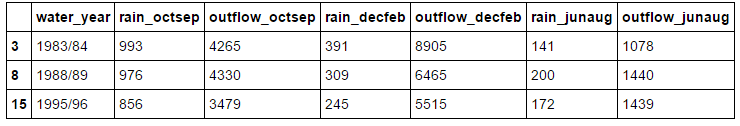```# Filtering by multiple conditionals
df[(df.rain_octsep < 1000) & (df.outflow_octsep < 4000)] # Can't use the keyword 'and'
```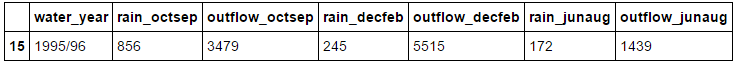```# Filtering by string methods
df[df.water_year.str.startswith('199')]
```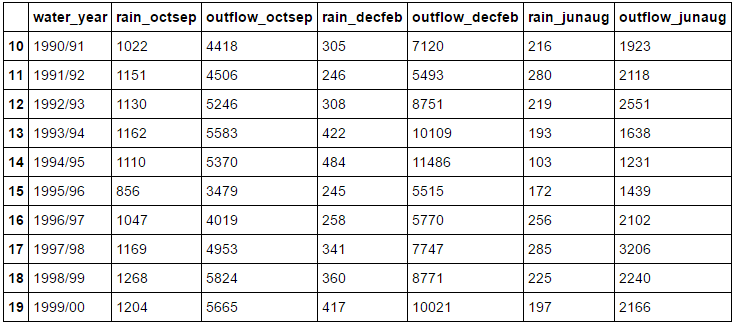## 索引

```# Getting a row via a numerical index
df.iloc
```

`iloc` 只对数字型的标签有用。它会返回给定行的 series，行中的每一列都是返回 series 的一个元素。

```# Setting a new index from an existing column
df = df.set_index(['water_year'])
```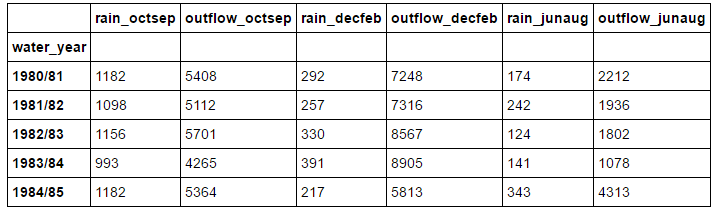```# Getting a row via a label-based index
df.loc['2000/01']
```

`iloc` 一样，`loc` 会返回你引用的列，唯一一点不同就是此时你使用的是基于字符串的引用，而不是基于数字的。

```# Getting a row via a label-based or numerical index
df.ix['1999/00'] # Label based with numerical index fallback *Not recommended
```

`iloc``loc` 一样，它也会返回你查询的行。

```df.sort_index(ascending=False).head(5) #inplace=True to apple the sorting in place
```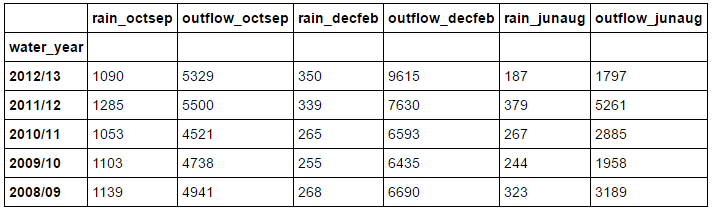```# Returning an index to data
df = df.reset_index('water_year')
```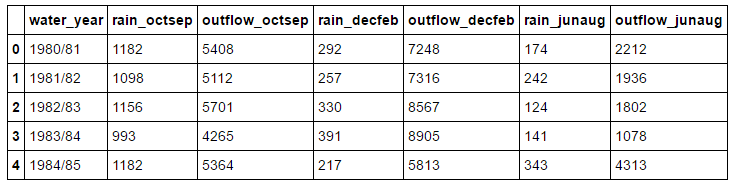## 对数据集应用函数

```# Applying a function to a column
def base_year(year):
base_year = year[:4]
base_year= pd.to_datetime(base_year).year
return base_year

df['year'] = df.water_year.apply(base_year)
```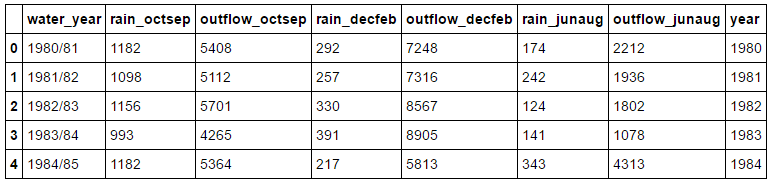## 操作数据集的结构

```#Manipulating structure (groupby, unstack, pivot)
# Grouby
df.groupby(df.year // 10 *10).max()
```

`groupby` 会按照你选择的列对数据集进行分组。上例是按照年代分组。不过仅仅这样做并没有什么用，我们必须对其调用函数，比如 `max``min``mean` 等等。例中，我们可以得到 90 年代的均值。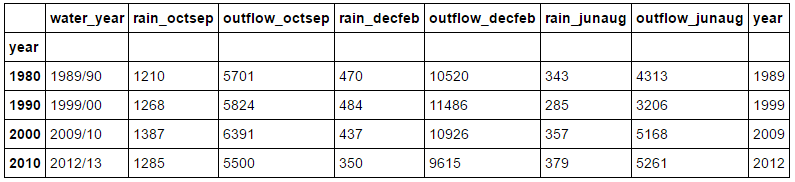```# Grouping by multiple columns
decade_rain = df.groupby([df.year // 10 * 10, df.rain_octsep // 1000 * 1000])[['outflow_octsep',                                                              'outflow_decfeb', 'outflow_junaug']].mean()
```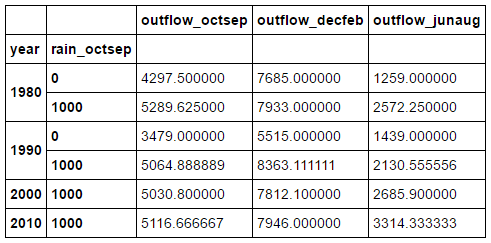```# Unstacking
```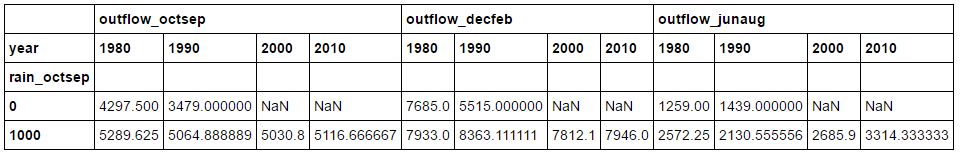```# More unstacking
``````# Create a new dataframe containing entries which
# has rain_octsep values of greater than 1250
high_rain = df[df.rain_octsep > 1250]
high_rain
```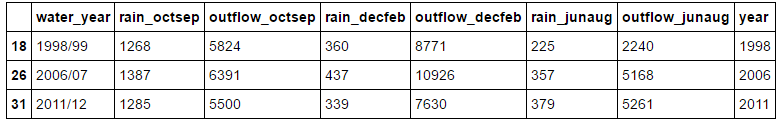```#Pivoting
#does set_index, sort_index and unstack in a row
high_rain.pivot('year', 'rain_octsep')[['outflow_octsep', 'outflow_decfeb', 'outflow_junaug']].fillna('')
```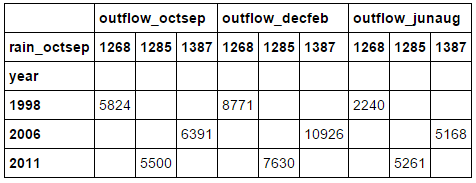## 合并数据集

```# Merging two datasets together
rain_jpn.columns = ['year', 'jpn_rainfall']

uk_jpn_rain = df.merge(rain_jpn, on='year')
```## 使用 Pandas 快速作图

`Matplotlib` 很棒，但是想要绘制出还算不错的图表却要写不少代码，而有时你只是想粗略的做个图来探索下数据，搞清楚数据的含义。Pandas 通过 `plot` 来解决这个问题：

```# Using pandas to quickly plot graphs
uk_jpn_rain.plot(x='year', y=['rain_octsep', 'jpn_rainfall'])
```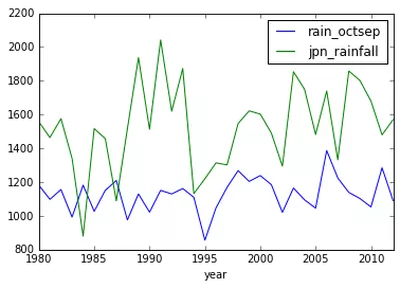## 保存你的数据集

```# Saving your data to a csv
df.to_csv('uk_rain.csv')
```

## 别忘了分享加关注

Python 翻译组是EarlGrey@编程派发起成立的一个专注于 Python 技术内容翻译的小组，目前已有近 30 名 Python 技术爱好者加入。